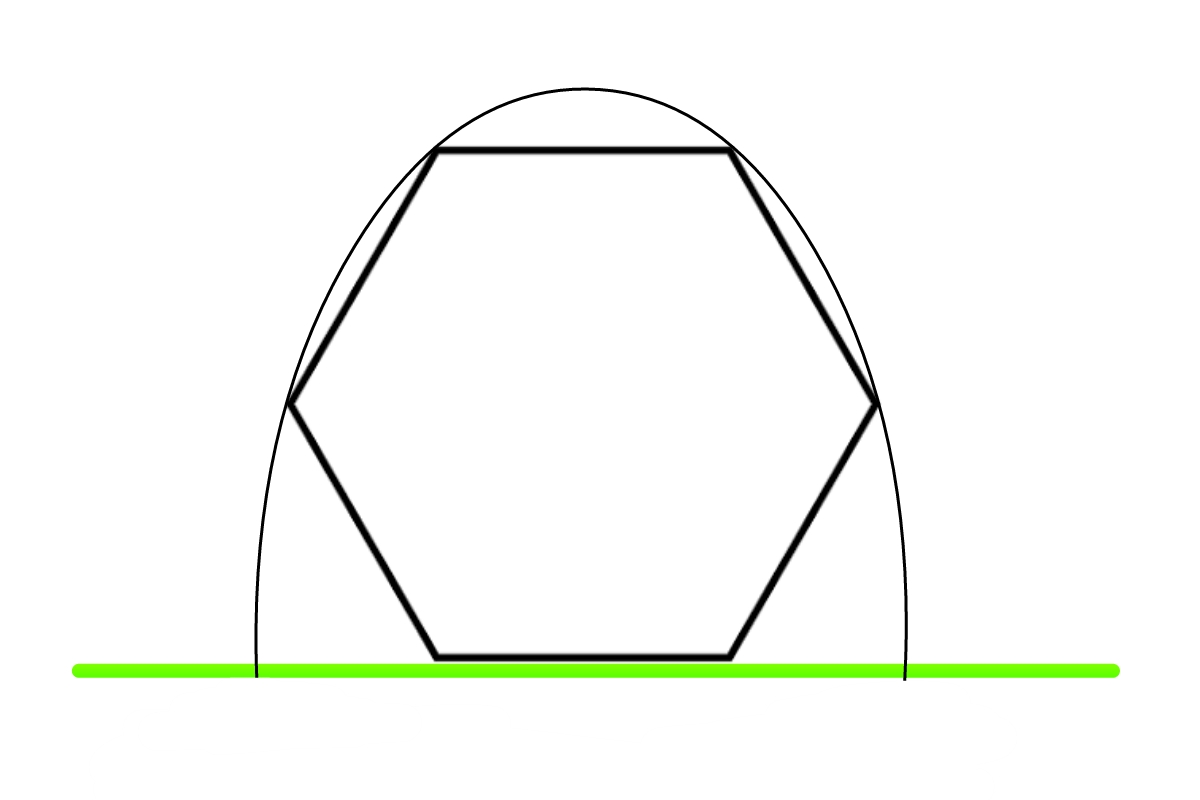# Grazing Through A Hexagon

Geometry Level 5A regular hexagon stands with one side on the ground and a particle is projected so as just to graze its four upper vertices. If the angle of projection can be written as $\arctan \left(a\sqrt{\dfrac{b}{c}} \right)$, where $a,b,c$ are positive integers, and $b,c$ are relatively prime and square-free. Find $a+b+c$.

×

Problem Loading...

Note Loading...

Set Loading...